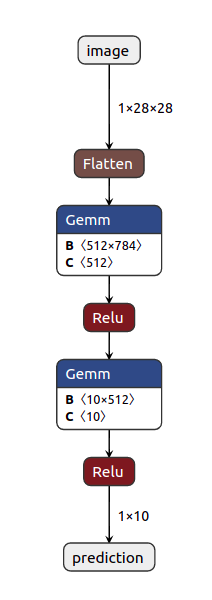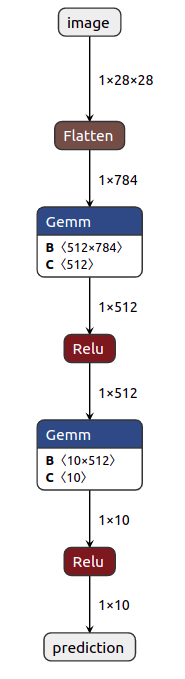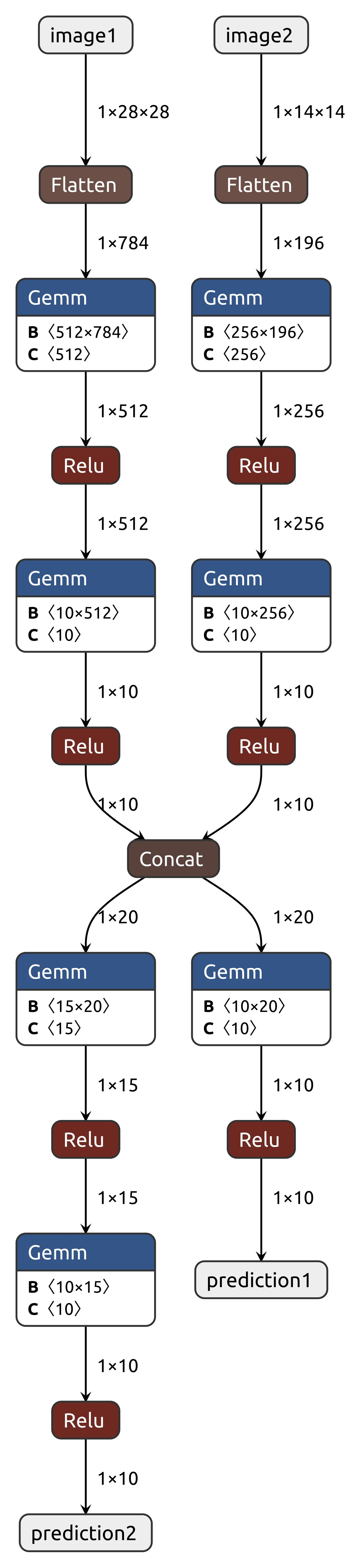# ML model visualization using Netron

## 1. Intro

• Netron is really great tool to visualize the ML models.
• ONNX (Open Neural Network Exchange Format) is a format designed to represent any type of Machine Learning and Deep Learning models.
• Pytorch and Tensorflow can export the model in onnx format.
• This onnx file can then be read with Netron to visualize the model graphically.

As simple as:

## 3. Barebone code

Output• Above code will open the visualization in browser @ `http://localhost:8080/`
• It needs a dummy input to pass through the model and calculate necessary data.
• Dummy input can be anything. It just needs to have right shape that model takes.
• To name the inputs and outputs, add following parameters to the `torch.onnx.export` function.## 4. Show tensor shapes at each step

• Install `onnx` package
• After exporting the onnx file, read back using `onnx.load` and then infer the tensor shapes

Output• This is a lot better visualization of model

## 5. Multiple Inputs Outputs

• Here is example using Two inputs and Two outputs

Output